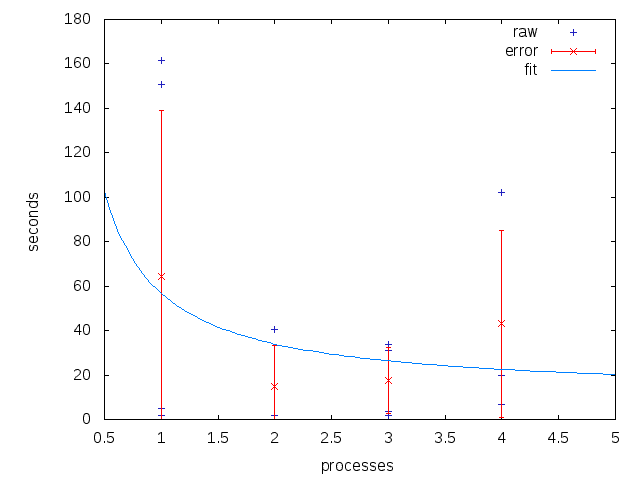Support via Liberapay, GitHub or PayPal

# Org Mode: Data Collection and Analysis

## Data Collection and Analysis

This example uses Org-babel to automate a repeated data-collection and analysis task. A Ruby code block is used to scrape data from the output of a computational experiment. This data is then written to an Org-mode table. A block of R code reads from this table and calculates lines of fit. Finally a block of gnuplot code is used to graph the results of both the raw data and the R analysis. By performing all of these steps within an Org-mode document working notes, discussion, and TODOs can be naturally interspersed with the code, and the results can easily be published to HTML or PDF for distribution.

### Requirement

• A working Ruby installation
• A working R installation
• A working gnuplot installation

• Org-babel handles passing the data between different programming languages.
• Raw data persists in tables in the Org-mode file.
• Working notes can be collocated with the code/results to which they refer.
• Tasks can be saved and updated from within the same file in which the work is being performed.
• Org-mode exporting facilities can be used to export the results to HTML or PDF for distribution.

• This approach can allow the experimenter to use whatever language is most comfortable for each sub-task, sometimes resulting in an overly complicated work flow. For example, in the example below I did not have to learn how to calculate the mean and standard deviation in R since it was easier for me to do so in Ruby even though a full R solution would have been more efficient.

### Example

#### Code for running experiment and collecting the results

This portion will not be repeatable as it would require the entire experimental setup. It is provided for demonstration.

Ruby run-timer-test: Runs the actual experiment. This is tangled to an external file and run on the command line – since these runs can take several days, I prefer to run them outside of Emacs (normally using screen).

```DEFAULT_CMDLINE = "--swap 0 --del 0 --mut 0.1 example.c "

def run_and_package(cmdline, package)
puts "#{package}: ../modify #{cmdline}"
start_time = Time.now
%x{../modify #{cmdline}}
total_time = Time.now - start_time
%x{echo "wall clock #{total_time}" >> gcd.c-.debug}
%x{rake package[#{package}]}
end

100.times do |n|
# run with default options
run_and_package(DEFAULT_CMDLINE, "normal_#{n}")
run_and_package("--pll_fit 2 "+DEFAULT_CMDLINE, "pll_2_#{n}")
run_and_package("--pll_fit 3 "+DEFAULT_CMDLINE, "pll_3_#{n}")
run_and_package("--pll_fit 4 "+DEFAULT_CMDLINE, "pll_4_#{n}")
run_and_package("--pll_fit 5 "+DEFAULT_CMDLINE, "pll_5_#{n}")
run_and_package("--pll_fit 6 "+DEFAULT_CMDLINE, "pll_6_#{n}")
run_and_package("--pll_fit 7 "+DEFAULT_CMDLINE, "pll_7_#{n}")
run_and_package("--pll_fit 8 "+DEFAULT_CMDLINE, "pll_8_#{n}")
end
```

Ruby parse-output: The execution of `run-timer-test` leaves results distributed across many text log files. The following Ruby source code block is used to collect results from these files and dump them into an Org-mode file as a table.

```def look(path)
processors = if path.match(/normal/)
"1"
elsif path.match(/pll_(\d+)_/)
\$1
else
0
end
generations =  results.match(/^Generations to solution: (\d+)/) ? Integer(\$1) : -1
total = results.match(/^ +TOTAL +([\d\.]+) /) ? Float(\$1) : -1
wall = results.match(/^wall clock ([\d\.]+)/) ? Float(\$1) : -1
fitness = results.match(/^ +fitness +([\d\.]+) +([\d\.]+) /) ? Float(\$2) : -1
mutation = results.match(/^ +mutation +([\d\.]+) +([\d\.]+) /) ? Float(\$2) : -1
[path, processors, total, wall, good_test, bad_test, compile, fitness, generations]
end

# puts "| path | processors | total | wall | fitness | mutation | generations |"
# puts "|-----------"

Dir.entries('./').select{|e| e.match(/[normalpll]+[_\d]+/)}.
map{|e| look(e)}.each{|row| puts "| "+row.join(" | ")+" |"}
```

#### Data

Here is fake example output from the `parse-output` Ruby source code block above.

 normal_0 1 150.264 150.631 163 1 pll_2_0 2 40.025 40.6989 39 3 pll_3_0 3 2.504 31.2146 2 1 normal_5 1 1.499 1.86636 2 2 pll_2_16 2 1.43 1.98515 1 1 normal_31 1 1.501 1.86745 2 1 pll_2_29 2 1.431 1.97831 1 1 normal_22 1 4.562 4.9299 3 3 pll_4_5 4 3.609 6.95303 4 1 normal_4 1 161.097 161.464 181 1 pll_3_3 3 1.751 33.8198 2 1 pll_4_2 4 99.546 102.202 72 2 pll_4_1 4 5.502 19.8754 3 1 pll_3_1 3 1.976 3.54056 2 2 pll_3_6 3 1.433 2.01857 1 1

#### Analysis

The code blocks in this section will be repeatable as they rely on the fake data given above.

Ruby calculate mean and standard deviation over the second column

```by_procs = {}
raw.each do |row|
by_procs[row] ||= []
by_procs[row] << row
end

by_procs.each do |key, vals|
mean = vals.inject(0){|sum, n| sum + n} / vals.size
stddev = Math.sqrt(vals.inject(0){|sum, n| sum + ((n - mean).abs * (n - mean).abs)} / vals.size)
puts "| #{key} | #{mean} | #{stddev} |"
end
```
 1 64.1518 75.1191 2 14.8875 18.2515 3 17.6484 14.907 4 43.0103 42.1863

R find the curve that best fits these data

```procs <- data\$V1
times <- data\$V2
df <- data.frame(procs, times)
nlsfit <- nls(times~c0 + (load/procs), data=df, start=list(load = 100, c0 = 20))
summary(nlsfit)
```
```Formula: times ~ c0 + (load/procs)

Parameters:
Estimate Std. Error t value Pr(>|t|)
c0      11.12      21.90   0.508    0.662

Residual standard error: 21.36 on 2 degrees of freedom

Number of iterations to convergence: 1
Achieved convergence tolerance: 3.924e-08
```

gnuplot plot the raw data, along with the error bars and the best fit curve.

```set xrange [0.5:5]
set yrange [0:]
set ylabel "seconds"
set xlabel "processes"
plot data using 2:4 with points title 'raw' linecolor 8
replot mydata using 1:2:3 with errorbars title 'error' linecolor 1
replot 11.12 + 45.70/x title 'fit'
```

Which produces the following#### Distribution

Using Org-mode's exporting capabilities it is easy to publish the entire working file including source-code and raw data, to share sections using `org-narrow-to-subtree', or even to share individual tables or graphs.

Documentation from the orgmode.org/worg/ website (either in its HTML format or in its Org format) is licensed under the GNU Free Documentation License version 1.3 or later. The code examples and css stylesheets are licensed under the GNU General Public License v3 or later.